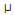# Electronics - Bipolar Junction Transistors (BJT) - Discussion

### Discussion :: Bipolar Junction Transistors (BJT) - General Questions (Q.No.14)

14.

If an input signal ranges from 20–40A (microamps), with an output signal ranging from .5–1.5 mA (milliamps), what is the ac beta?

 [A]. 0.05 [B]. 20 [C]. 50 [D]. 500

Explanation:

No answer description available for this question.

 Rupali said: (Feb 26, 2011) Beta = (1.5-.5)mA/(40-20)micro Amp = 50

 Fredy And Vimal said: (Jun 15, 2011) beta=d(Ic)/d(Ib) typical value 50 - 250

 Kosgei said: (Jul 27, 2011) Ensure you change the micro ampheres to milliamphere.

 Vinod Kumar said: (Aug 30, 2011) Beta=D(output)/D(input) => Beta= (1.5-0.5)*10^-3/(40-20)*10^-6 => Beta= 1*10^3/20 => Beta= 1000/20 => Beta= 50

 Nidhi Patel said: (Jan 6, 2014) Beta = (1.5-0.5)*10^-3/(40-20)*10^-6. you can get answer by solving above expression.

 Jasmine George said: (May 28, 2014) Beta = current gain = output current/input current. = ((1.5*10^-3)-(.5*10^-3)/(40*10^-6)-(20*10^-6)). = 50.

 Dishant Garg said: (Aug 11, 2014) Beta = (collector current gain)/(base current gain). = (1*0.001)/20*0.000001. = 50.

 Jitesh said: (Mar 3, 2019) Beta is output /input. i.e (1.5-0.5)/(40-20).

 Tkay said: (Aug 12, 2019) 1*10^-3/20*10^-6 = 50. From equation B=collector current gain /base current gain.